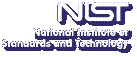Up# First and Last Passage Random Walk Algorithms.

Michael Mascagni
Department of Computer Science, Florida State University

Monday, December 10, 2001 15:00-16:00,
Room 145, NIST North (820)
Gaithersburg
Monday, December 10, 2001 13:00-14:00,
Room 4511
Boulder

Abstract: We present two complimentary Monte Carlo methods for computing the charge density on a conductor when that conductor is at potential V0 with respect to infinity.The first method extends our previous first-passage algorithm for calculating the capacitance of an arbitrarily shaped conducting object.  The capacitance of an object can be probabilistically calculated finding the probability that a Brownian walker starting at infinity hits the region before returning to infinity.  It is a standard result from probabilistic potential theory that the absorption locations of the first-passage capacitance calculation give the charge distribution on the conducting object. The second Monte Carlo method utilizes the last-passage concept. This last-passage method stems from the consideration of the isomorphism between the electrostatic potential and the probability of going to infinity without touching a conducting object at a certain distance from the conductor. This is analogous to `adjoint' methods, where time-reversedwalks are used to solve the adjoint problem. We demonstrate our methods for computing an analytically known charge density on a two dimensional thin disk in three dimensions. In addition we solve for the charge distribution on the unit cube using an edge distribution concept. Computing the capacitance of the unit cube is considered the most important open problem in electrostatics, and we not only have the most accurate calculation, but the edge distribution computation provides results never before computed.
Contact: R. F. Boisvert

Note: Visitors from outside NIST must contact Robin Bickel; (301) 975-3668; at least 24 hours in advance.